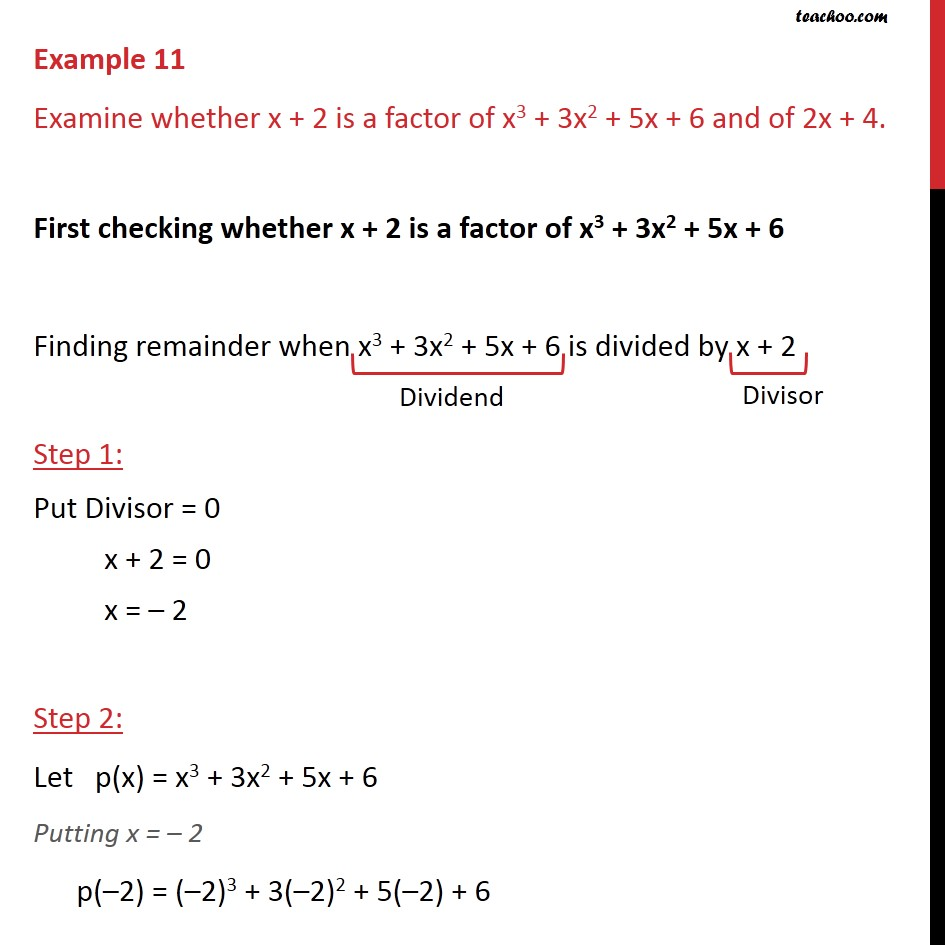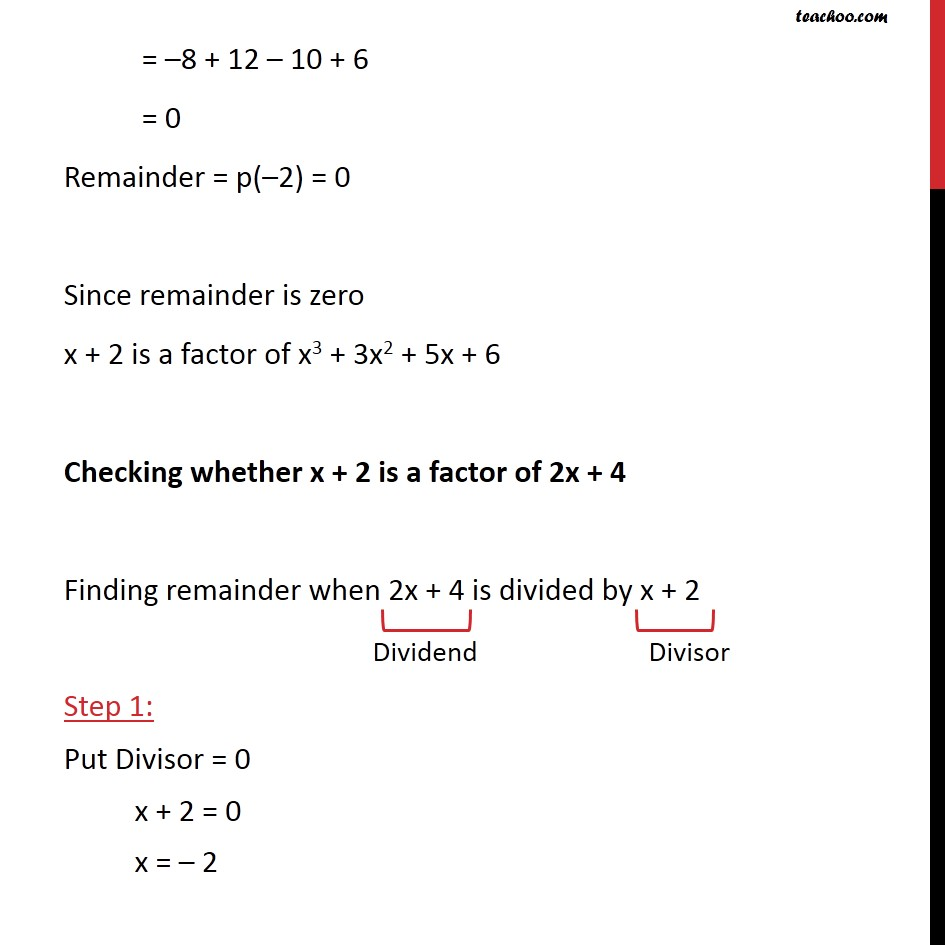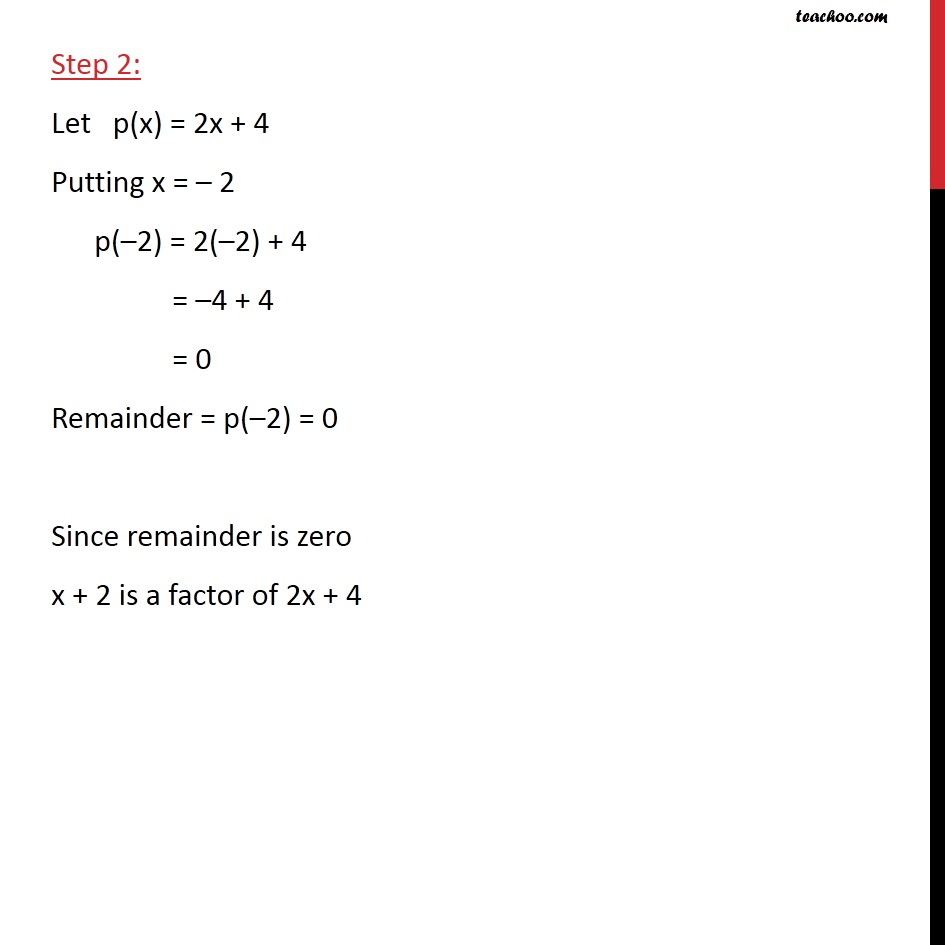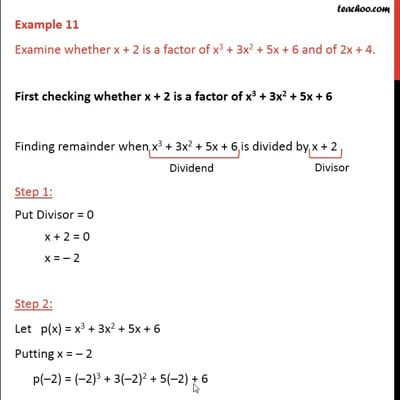Examples

Chapter 2 Class 9 Polynomials
Serial order wiseThis video is only available for Teachoo black users

Learn in your speed, with individual attention - Teachoo Maths 1-on-1 Class

### Transcript

Example 6 Examine whether x + 2 is a factor of x3 + 3x2 + 5x + 6 and of 2x + 4. First checking whether x + 2 is a factor of x3 + 3x2 + 5x + 6 Finding remainder when x3 + 3x2 + 5x + 6 is divided by x + 2 Step 1: Put Divisor = 0 x + 2 = 0 x = 2 Step 2: Let p(x) = x3 + 3x2 + 5x + 6 Putting x = 2 p( 2) = ( 2)3 + 3( 2)2 + 5( 2) + 6 = 8 + 12 10 + 6 = 0 Remainder = p( 2) = 0 Since remainder is zero x + 2 is a factor of x3 + 3x2 + 5x + 6 Checking whether x + 2 is a factor of 2x + 4 Finding remainder when 2x + 4 is divided by x + 2 Step 1: Put Divisor = 0 x + 2 = 0 x = 2 Step 2: Let p(x) = 2x + 4 Putting x = 2 p( 2) = 2( 2) + 4 = 4 + 4 = 0 Remainder = p( 2) = 0 Since remainder is zero x + 2 is a factor of 2x + 4